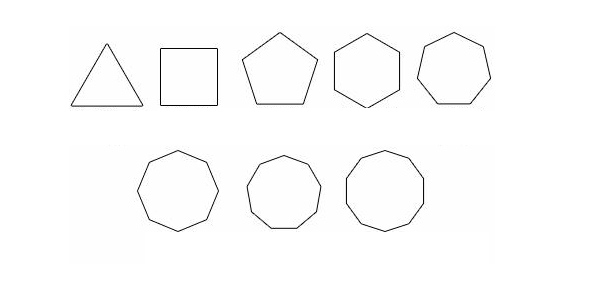# Polygon And Polyhedra

6 Questions | Total Attempts: 112SettingsQuiz on polyhedra shapes and their properties. Also review questions on area and perimeter of polygons.

Related Topics
• 1.
If an object has 8 vertices, 12 edges and 6 faces, what possible polyhedra could it be?
• A.

Rectangular prism and cylinder

• B.

Cube and cone

• C.

Cube and rectangular prism

• D.

Square pyramid and triangular prism

• 2.
Which object has two circle bases and a curved body?
• A.

Cone

• B.

Cylinder

• C.

Sphere

• D.

Prism

• 3.
To find the area of a rectangle, you
• A.

Multiple base and height

• B.

Multiple base and height and divide by 2.

• C.

Add the two bases together, then multipy by the height then divide by 2.

• D.

None of the above

• 4.
To find the area of a triangle, you must
• A.

Multiply base and height.

• B.

Multiply base and height then add the sides.

• C.